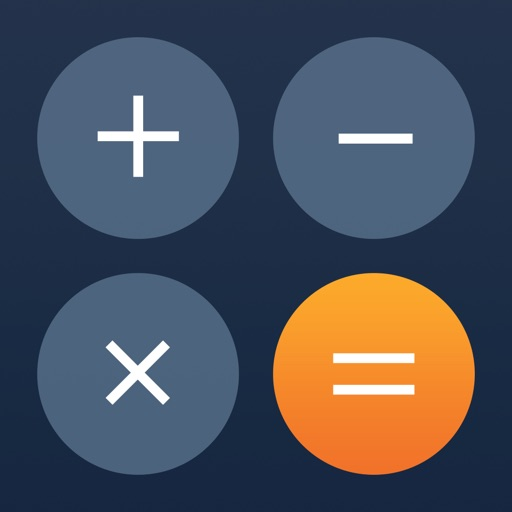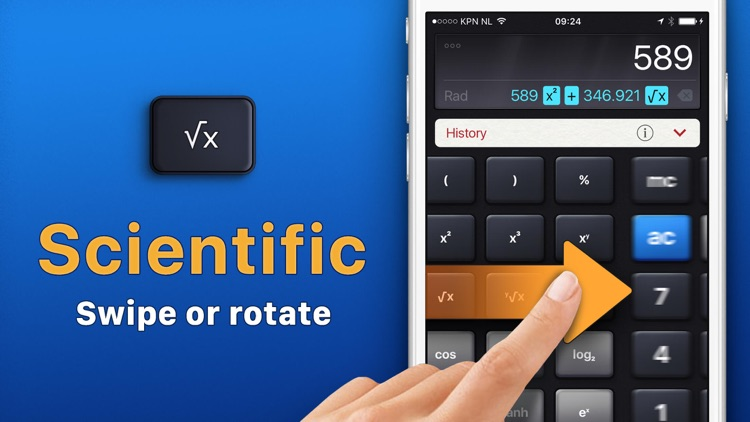## Count on Calculator HD Ad-Free: Simple and Powerful, with big buttons for daily calculations# Calculator HD

by Impala StudiosCount on Calculator HD Ad-Free: Simple and Powerful, with big buttons for daily calculations. Start Calculating Now! Calculator HD combines the Standard & Scientific calculator, with extensive & easy functionality options. Use the new Retro Theme and go vintage. ; )### App Details

Version
3.5.12
Rating
(13161)
Size
108Mb
Genre
Utilities Education
Last updated
April 19, 2021
Release date
December 21, 2011

### App Screenshots### App Store Description

Count on Calculator HD Ad-Free: Simple and Powerful, with big buttons for daily calculations. Start Calculating Now! Calculator HD combines the Standard & Scientific calculator, with extensive & easy functionality options. Use the new Retro Theme and go vintage. ; )

Free in Calculator HD
• Basic & Scientific Calculations
• Vintage Calculator Retro Theme
• History Tape: Copy & Share
• Edit Equation
• Calculate with Degrees or Radians
• Memory
• Set the Number of Decimals
• Dark Mode
• Support for iPad Split View

>> Have any questions?
If you are unable to find the answers to your questions, our support team is ready and happy to help at [email protected]!

EDIT EQUATION
You can edit both digits and operators of the current equation.

Mathematical Operations
Addition, Subtraction, Division, Multiplication, Percentages, Square, Cubic Exponent, Nth Exponent, Square Root, Cubic Root, Nth Root, Engineering Exponent, Factorial, Power of Two, Power of Ten, Power of E, Natural Logarithm, Common Logarithm, Binary Logarithm, Sine, Cosine, Tangent, Arc Sine, Arc Cosine, Arc Tangent, Hyperbolic Sine, Hyperbolic Cosine, Hyperbolic Tangent, Hyperbolic ArcSine, Hyperbolic Arc Cosine, Hyperbolic ArcTangent, Multiplicative Inverse, Random Number Input